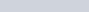ChemTalk

# SI Units## Core Concepts

Standard International (SI) Units are the basis for all physical measurements, and therefore have an incredible importance in science today. In this article, we describe the base units, the derived units, and some important non-SI units.

## What Are SI Units?

The International System of Units (SI), sometimes referred to as the metric system, is the international measuring standard. The SI Units serve as a consistent way of communicating measurements like temperature, mass, and distance. It allows people from all over the world to be able to speak the same language when discussing physical quantities.

## The Seven Base Units

The SI Units system is based around seven base units:

• seconds for measuring time
• meter for measuring length
• kilogram for measuring mass
• ampere for measuring electric current
• Kelvin for measuring temperature
• mole for measuring amount of a substance
• candela for measuring luminous intensity

### The Derived Units

A derived unit is a unit of measurement derived from the seven SI base units. Derived units may not have dimensions or are a combination of base units. For example, the unit for area is a derived unit. To calculate area you multiply length by width, a distance by a distance, therefore the unit is a square meter (m2).

##### Named Derived Units

There are a total of 22 derived units with special names.

##### Unnamed Derived Units

Mathematical combinations of SI base units make up many more derived units that don’t have special names.

## The Accepted Non-SI Units

There are also a number of units in the metric system that are neither base units nor derived units. Because they are multiples or fractions of SI units or because they are practical, these units are included in the metric system. Accepted units are units that can be used with the SI Units. In other words, accepted units are accepted in most journals and research papers but are not base units of the SI system.

## SI Units Practice Problems

Problem 1

An imprecise physicist might call a certain derived unit a “newton meter per second”. What derived unit would he or she therefore be talking about?

Problem 2

The Ideal Gas Law has the following equation:• P is for pressure
• V is for volume
• n is for moles
• T is for temperature
• R is a constant

What base SI units are involved in the Ideal Gas Law? (i.e. not just the derived units)

## SI Units Practice Problem Solution

1. The Watt
2. kg, m, s, mol, K